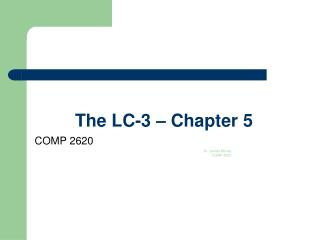DownloadDownload PresentationThe LC-3 – Chapter 5

# The LC-3 – Chapter 5

Download Presentation## The LC-3 – Chapter 5

- - - - - - - - - - - - - - - - - - - - - - - - - - - E N D - - - - - - - - - - - - - - - - - - - - - - - - - - -
##### Presentation Transcript

1. The LC-3 – Chapter 5 COMP 2620 Dr. James Money COMP 2620

2. Operate Instructions • Recall that there are three types of instructions: • Operate instructions • Data movement instructions • Control instructions • We first consider the operate instructions today

3. Operate Instructions • Remember these types of instructions process data • It includes the arithmetic operations such as ADD, SUB, MUL, DIV • In addition, it includes the logical instructions such as AND, OR, NOT, XOR

4. Operate Instructions • The LC-3 has three operate instructions • ADD • AND • NOT • We will have to essentially emulate the remaining operations that we need

5. Operation: NOT • The NOT instruction is the only operate instruction that is unary - it requires only one source operand • The opcode is 1001 • The output of the operation is the 16-bit logical NOT of the source operand • NOT uses register addressing mode

6. Note: The source and destination operands can be the same

7. Operation: NOT • Consider the instruction: 1001 011 101 111111 • SRC = 101 = R5 • DST = 011 = R3 • Assume R5=1010 1111 0000 1111Then, after the instruction R3=0101 0000 1111 0000

8. Operation: NOT

9. Operations: ADD and AND • These are a binary operations – they require two inputs • The opcodes are • ADD – 1001 • AND - 0101 • The source operands are 16 bits • The first operand is a register

10. Operations: ADD and AND • ADD performs a two’s complement addition of the two source operands • AND performs a bitswise AND operation on the two source operands • The second is either a register or immediate value • Bit = 0 implies register • Bit = 1 implies immediate mode

11. Register Mode this zero means “register mode”

12. Immediate Mode this one means “immediate mode” Note: Immediate field issign-extended.

13. Operations: ADD and AND • Assume R4=6, R5=-18 • Consider the instruction 0001 001 100 000 101 • Then, R1=-12 • That is R1= R4+R5